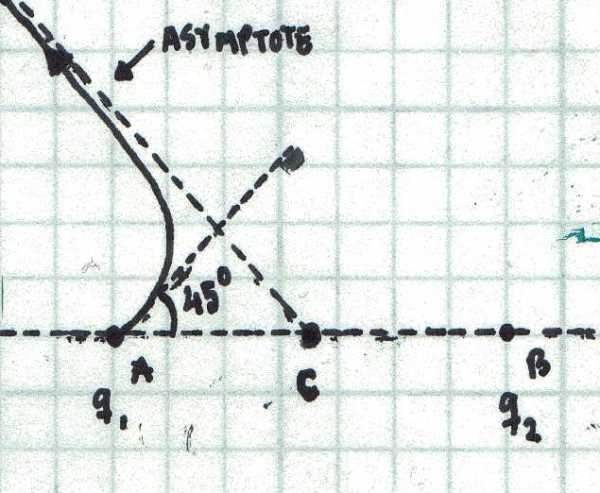# Asymptotic Field LinesConsider two charges $$q_{1}=3~\mu\text{C}$$ and $$q_{2}=1~\mu \text{C}$$ located at points A and B, separated by a distance $$\overline{AB}=10~\text{cm}$$. Since both charges have the same sign, any field emanating from one of the charges will go off to infinity. It is clear, that far away from the charges, the field lines become straight lines, and therefore, they have oblique asymptotes. Consider a field line exiting the charge $$q_{1}$$ at $$\alpha=45^{\circ}$$ (as shown in the figure). Let C denote the point where the oblique asymptote corresponding to this line intersects $$\overline{AB}$$. Determine the distance $$\overline{AC}$$ in centimeters.

×

Problem Loading...

Note Loading...

Set Loading...